## Chapter Goals

• To be able to write programs with standard lists and iterators
• To understand the advantages and disadvantages of the list data structure
• To be able to implement linked lists
• To learn about stacks and queues
• To become familiar with common containers and algorithms from the Standard Template Library

• Suppose you are maintaining a vector of sorted objects (such as employees)
• Many elements will need to be shifted back if an new object is inserted in the middle.
• Many elements will need to be shifted forward if an object is deleted from the middle.
• Moving a large number of records can involve a substantial amount of computer time.
• Rather than store a sequence of values in one long block of memory (like a vector or an array) a linked list stores each value in its own memory block, together with the locations of the neighboring blocks in the sequence.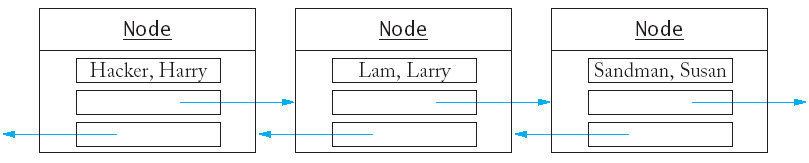• Inserting an element into a list now requires no shifting, merely reassigning new locations to adjacent objects.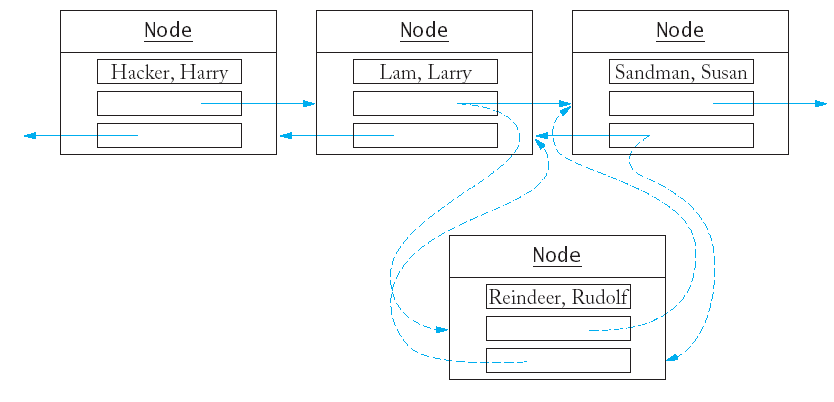• Removing an element from the list doesn't require shifts either.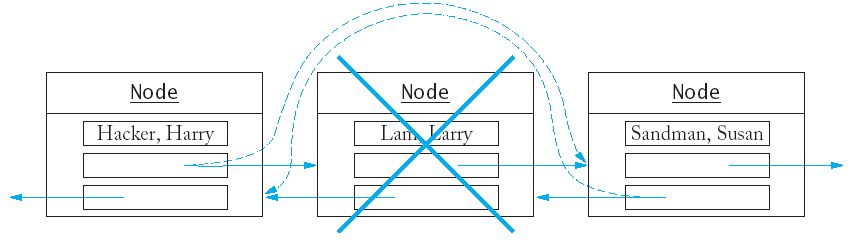• The standard C++ library has an implementation of the linked list structure.
• First we will learn how to use the standard list.
• Later we will find out how to implement lists.
• Doubly linked lists (as shown in the illustrations) have links going in both directions.
• Just like the vector, the standard list is a template.
• You can use push_back to add elements to the list.
`list<string> staff;staff.push_back("Cracker, Carl");staff.push_back("Hacker, Harry");staff.push_back("Lam, Larry");staff.push_back("Sandman, Susan");`
• You cannot directly access elements using subscript access (e.g. staff) - the values are not stored in one contiguous block in memory and there is no fast way to access them.
• Instead you must start at the beginning of the list, and visit each element in turn using a list iterator. An iterator marks a position in the list.
`list<string>::iterator pos;pos = staff.begin();`
• To move an iterator forward in the list, use the ++ operator.
`pos++;`
• To move an iterator backward in the list, use the -- operator.
`pos--;`
• You can find the value that is stored in the position with the * operator.
`string value = *pos;`
• The value *pos represent the value that is stored in the list.
`*pos = "Van Dyck, Vicki"; // assign a value pos = staff.begin(); // assign a position   `
• To insert another string before the iterator position, use the insert function.
`staff.insert(pos, "Reindeer, Rudolph");`
• Each list has an end position that does not correspond to any value in the list but that points past the list's end.
`pos = staff.end(); /* points past the end of the list */staff.insert(pos, "Yaglov, Yvonne");   /* insert past the end of list */`
• The end position does not point to any value, so you cannot look up the value at that position.
`string value = *(staff.end()); /* ERROR */`
• Compare to accessing s in a vector with 10 elements.
• The end position is useful for stopping a traversal of the list.
`pos = staff.begin();while (pos != staff.end()){  cout << *pos << "\n";   pos++;}`
• The traversal can be described more concisely with a for loop:
`for (pos = staff.begin(); pos != staff.end(); pos++)   cout << *pos << "\n";`
• Compare this to a traversal of a vector.
`for (i = 0; i < s.size(); i++)   cout << s[i] << "\n";`
• To remove an element from a list, you move an iterator to the position you want to remove, then call the erase function.
`pos = staff.begin();pos++;staff.erase(pos);`

## Implementing Linked Lists (The Classes for Lists, Nodes, and Iterators)

• A linked list stores each value in a separate object called a node.
`class Node {public:   Node(string s);private:   string data;   Node* previous;   Node* next;friend class List;friend class Iterator;};`
• The friend declarations indicate the List and Iterator member functions are allowed to inspect and modify the data members of the Node class.
• A class should not grant friendship to another class lightly, because it breaks the privacy protection.
• The list object holds the locations of the first and last nodes in the list.
`class List {public:   List();   void push_back(string s);   void insert(Iterator pos, string s);   Iterator erase(Iterator pos);   Iterator begin();   Iterator end();private:   Node* first;   Node* last;};`
• When the list is empty the first and last pointers are NULL.
• A list object stores no data; it just knows where to find the node objects that store the list contents.
• An iterator denotes a position in the list.
`class Iterator {public:   Iterator();   string get() const;     /* use instead of * */   void next();            /* use instead of ++ */   void previous();        /* use instead of -- */   bool equals(Iterator b) const; /* use instead of == */private:   Node* position;   Node* last;friend class List;};`
• We will enable the operators ++, --, * and == in the next chapter.
• If the iterator points past the end of the list, then the position pointer is NULL.
• The iterator stores a pointer to the last element of the list, so that the previous method can move the iterator back from the past-the-end position to the last element of the list.

## Implementing Linked Lists (Implementing Iterators)

• Iterators are created by the begin and end functions of the List class.
`Iterator List::begin() {   Iterator iter;   iter.position = first;   iter.last = last;   return iter;}Iterator List::end() {   Iterator iter;   iter.position = NULL;   iter.last = last;   return iter;}`
• The next function advances the iterator to the next position.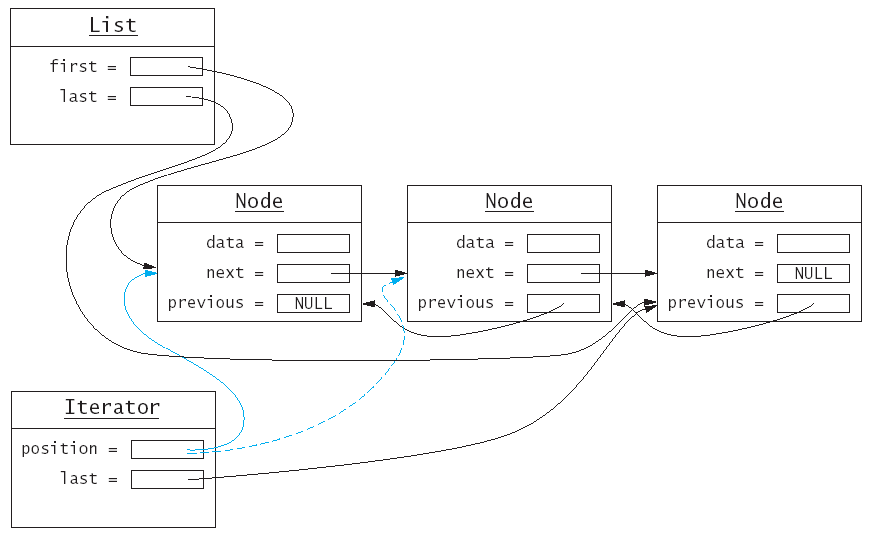• Note that it is illegal to advance the iterator once it is in the past-the-end position.
`void Iterator::next(){  assert(position != NULL)   position = position->next;}`
• The previous function is similar.
• When the iterator points to the first element in the list, it is illegal to move it further backward.
`void Iterator::previous(){  if (position == NULL) position = last;   else    position = position->previous;   assert(position != NULL);}`
• The get function simply returns the data value of the node to which position points.
`string Iterator::get() const{  assert(position != NULL);   return position->data;}`
• The equals function compares two position pointers.
`bool Iterator::equals(Iterator b) const{  return position == b.position; }`

## Implementing Linked Lists (Implementing Insertion and Removal)

• For the push_back function, we must first make a new node.
`Node* newnode = new Node(s);`
• The node must point back to the old end of the list, while the old end must point to it.
`newnode->previous = last; // (1)last->next = newnode;     // (2)last = newnode;           // (3) !`
• There is a special case when last is NULL (the list is empty).
`if (last == NULL){  first = newnode;   last = newnode;}`
• The complete member-function:
`void List::push_back(string s){  Node* newnode = new Node(s);   if (last == NULL) /* list is empty */   {  first = newnode;      last = newnode;   }   else   {  newnode->previous = last;      last->next = newnode;      last = newnode;   }}`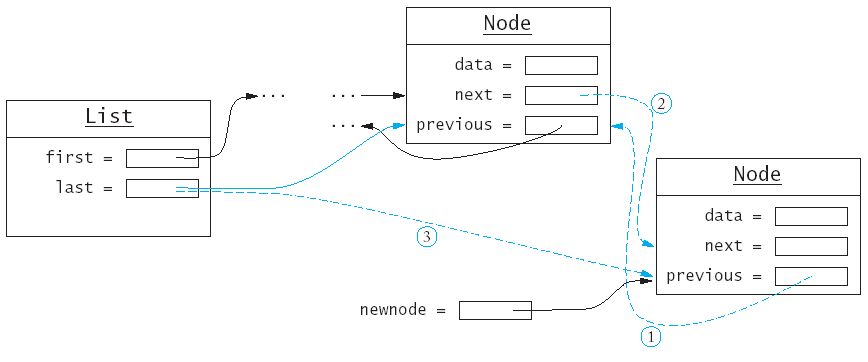• To insert an element in the middle of the list requires manipulating both elements on either side of the new node.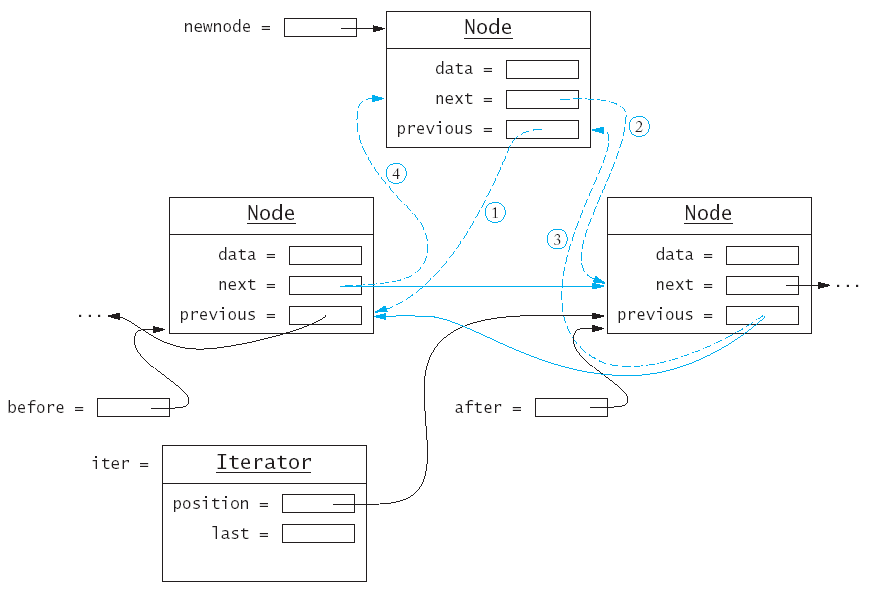• There is a special case when the list is empty.
`if (iter.position == NULL){  push_back(s);   return;}`
• Otherwise we create pointers to track the surrounding nodes.
`Node* after = iter.position;Node* before = after->previous;`
• We tell the new node where it belongs in the list.
`newnode->previous = before; // (1)newnode->next = after;      // (2)`
• Then update the surrounding nodes.
`after->previous = newnode;  // (3)if (before == NULL) /* insert at beginning */   first = newnode;else   before->next = newnode;  // (4)`
• When know that after is not NULL, but before could be.

• As with insertion, we will need to work with both the element before and after the one to be removed.Of course we cannot remove a node that is not there.

`assert(iter.position != NULL)`

We create pointers to track all three nodes we need to work with.

`Node* remove = iter.position;Node* before = remove->previous;Node* after = remove->next;`

We disconnect the node to be removed from the one before it; note the special case when we delete from the front of the list.

`if (remove == first) first = after;else   before->next = after;`

We repeat the process with the node after the one to delete.

`if (remove == last) last = before;else   after->previous = before;`

Finally we delete the node.

`delete remove;`

## Stacks and Queues

• Stacks and queues are special data types that allow insertion and remove of items at the ends only, not in the middle.
• A stack lets you insert and remove elements at one end only, traditionally called the top of the stack.
• To visualize a stack, think of a stack of books.
• Since items can only be added or removed from the top of the stack, they are removed in the order that is opposite from the order they were added.
• This is called last in, first out or LIFO order.
• The addition and removal operations are called push and pop.
• The is a stack template in the standard C++ library.
`stack<string> s;s.push("Tom");s.push("Dick");s.push("Harry");while (s.size() > 0){  cout << s.top() << "\n";   s.pop();}`
• A queue is similar to a stack, except that you add items to one end of the queue (the back) and remove them from the other end of the queue (the front).
• To visualize a queue, think of people lining up.
• Queues store items in a first in, first out or FIFO fashion.
• The standard queue template implements a queue in C++.
`queue<string> q;q.push("Tom");q.push("Dick");q.push("Harry");while (q.size() > 0){  cout << q.front() << "\n";   q.pop();}`

## Other Standard Containers

• The standard library contains several other useful containers.
• The set always keeps its elements in order, no matter in which order you insert them.
`set<string> s;s.insert("Tom");s.insert("Dick");s.insert("Harry");set<string>::iterator p;for (p = s.begin(); p!= s.end(); p++)   cout << *p << "\n";`
• The above code displays the strings in sorted order: Dick, Harry, Tom.
• The set data structure keeps the values in a special tree shaped structure; each time an element is inserted, the tree is reorganized.
• The C++ set ignore duplicates; if you insert an element into the set that is already present, the insertion has no effect.
• The count function returns the number of times that an element is contained in a set (should always be 1).
`set<string> s;s.insert("Tom");s.insert("Tom");cout << s.count("Tom") << "\n"; /* displays 1 */`
• If you want to be able to keep track of multiple occurrences of identical values, use a multiset instead.
• The count function works for multiset, with expected results.
`multiset<string> m;m.insert("Tom");m.insert("Tom");cout << m.count("Tom") << "\n"; /* displays 2 */`
• A map is similar to a vector, but you can use another data type for the indices.
`map<string, double> scores;scores["Tom"] = 90;scores["Dick"] = 86;scores["Harry"] = 100;`

## Standard Algorithms

• Why iterators?
• It is possible to supply generic functions that can carry out a task with the elements in any container that uses iterators.
• Example: The accumulate function can compute the sum of all elements in a vector or a list.
`vector<double> data;/* do something with data */double vsum = 0;accumulate(data.begin(), data.end(), vsum);/* now vsum contains the sum of the elements in the vector */list<double> salaries;/* do something with salaries */double lsum = 0;accumulate(salaries.begin(), salaries.end(), lsum);/* now lsum contains the sum of the elements in the list */`
• The standard library also supplies a find algorithm.
• The find algorithm returns a position or the second iterator (from the parameter list) if the search fails.
`/* search for a certain name on the staff */list<string>::iterator it =   find(staff.begin(), staff.end(), name);`
• The first two parameters can be other iterators for the list (if you don't want to search the entire list).
• The standard library provides a wealth of ready-to-use and fully debugged data structures and algorithms.
• for_each applies a function to each element
• find (as above)
• find_if locates the first element fulfilling a condition
• count (as above)
• equal tests if containers have the same elements in the same order
• replace/replace_if replace all matching elements with a new one
• unique remove adjacent identical values
• min_element, max_element finds the smallest and largest elements
• next_permutation rearranges the elements; call it n! times iterates through all permutations
• sort sorts the elements; stable_sort performs better if the container is already almost sorted
• random_shuffle randomly rearranges the elements
• nth_element find the nth element without sorting the container.
• plus many more...
• Before writing a lot of code from scratch, check whether the standard library already has what you need.